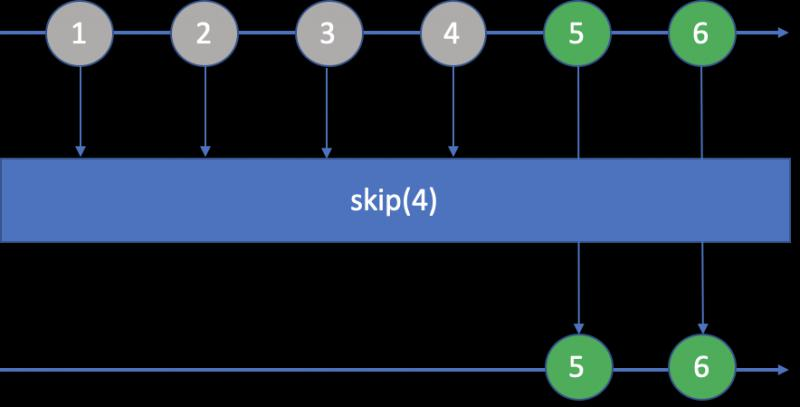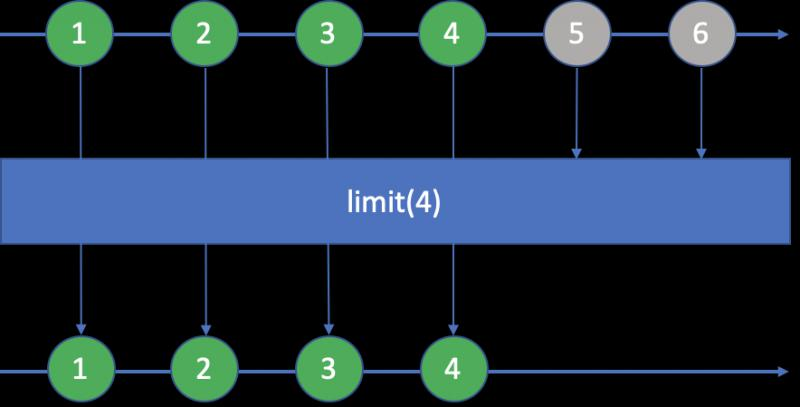# 2 skip()方法``````List<Integer> result = Stream.of(1, 2, 3, 4, 5, 6)
.skip(4)
.collect(Collectors.toList());
List<Integer> expected = asList(5, 6);
assertEquals(expected, result);
``````

（1）当`n<0`时，抛`IllegalArgumentException`异常；

（2）当`n=0`时，相当没有跳过任何元素，原封不动、完璧归赵；

（3）当`0<n<length`时，跳过n个元素后，返回含有剩下的元素的流；

（4）当`n>=length`时，跳过所有元素，返回空流。

# 3 limit()方法``````List<Integer> result = Stream.of(1, 2, 3, 4, 5, 6)
.limit(4)
.collect(Collectors.toList());
List<Integer> expected = asList(1, 2, 3, 4);
assertEquals(expected, result);
``````

（1）当`n<0`时，抛`IllegalArgumentException`异常；

（2）当`n=0`时，不取元素，返回空流；

（3）当`0<n<length`时，取前n个元素，返回新的流；

（4）当`n>=length`时，取所有元素，原封不动、完璧归赵。

# 4 对无限流的操作

``````Stream.iterate(1, i -> i + 1)
.filter(num -> (num & (num - 1)) == 0)
.limit(10)
.forEach(System.out::println);
System.out.println("----------------");
Stream.iterate(1, i -> i + 1)
.filter(num -> (num & (num - 1)) == 0)
.skip(10)
.forEach(System.out::println);
``````

1, 2, 4, 8, 16, 32, 64, 128, 256, 512

`skip()`而跳过了前10个，继续输出，但会不断执行下去（会有int的溢出现象）：

1024, 2048, 4096, 8192, 16384, 32768...

# 5 组合应用

## 5.1 与subList的替换

``````List<Integer> list = asList(1, 2, 3, 4, 5, 6, 7, 8, 9);
List<Integer> expected = list.subList(3, 7);

List<Integer> result = list.stream()
.skip(3)
.limit(7 - 3)
.collect(Collectors.toList());
assertEquals(expected, result);
``````

`subList(startIndex, endIndex)`转换成`skip(startIndex).limit(endIndex - startIndex)`

## 5.2 分页

``````int pageSize = 10;
int pageIndex = 7;

List<Integer> expected = asList(61, 62, 63, 64, 65, 66, 67, 68, 69, 70);
List<Integer> result = Stream.iterate(1, i -> i + 1)
.skip((pageIndex - 1) * pageSize)
.limit(pageSize)
.collect(Collectors.toList());

assertEquals(expected, result);
``````

# 6 总结